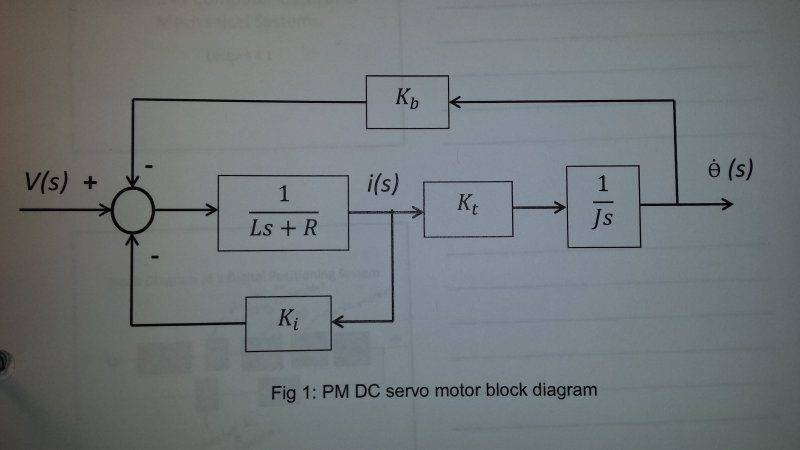# Transfer Function of Block Diagram

• ThLiOp
In summary, the conversation discusses the block diagram of a PM DC servo motor with current loop feedback and the approximation of its transfer function. The simplified transfer function is shown to be G(s) = Kt / (JLs2 + J(R + Ki)s + KtKb), and the attempt to factor the denominator using the quadratic formula is also mentioned. However, it is noted that the final transfer function presented in the homework does not match the one obtained through this method.

## Homework Statement

The block diagram of a PM DC servo motor with current loop feedback is shown below:If Ki is adjusted such that J(Ki+R)2 >> 4KTKbL, show that the transfer function may be approximated by

G(s) = (1/Kb) / (τms+1)(τes+1),

where

τm = J(R+Ki) / KTKb

τe = L / (R+Ki)

## The Attempt at a Solution

[/B]
I simplified the block diagram and got the transfer function:

G(s) = Kt / (JLs2 + J(R + Ki)s + KtKb)

Then I tried to factor the denominator. When using the quadratic formula, I found:

(-JR - JKi) +/- sqrt( J[ J(R+Ki)2 - 4KtKbL]) / 2JL

Assuming the conditions presented for Ki, I canceled out the 4KtKbL.

Am I on the right path? In the end, I still couldn't get the transfer function presented in the homework.

ThLiOp said:
I simplified the block diagram and got the transfer function:

G(s) = Kt / (JLs2 + J(R + Ki)s + KtKb)
That seems fine.

ThLiOp said:
Then I tried to factor the denominator. When using the quadratic formula, I found:

(-JR - JKi) +/- sqrt( J[ J(R+Ki)2 - 4KtKbL]) / 2JL
Those are just the roots, though. If ##ax^2 + bx + c## is your polynomial, then its factored form is ##a(x - x_1)(x - x_2)##, where ##x_1## and ##x_2## are its roots.

ThLiOp said:
Am I on the right path? In the end, I still couldn't get the transfer function presented in the homework.
I can't either. Going your route, and I think the approximation shown is highly suggestive of that, I get the form:
$$\frac{\dot{\theta}(s)}{V(s)} = \frac{\frac{1}{K_b}}{\tau_m s (\tau_e s + 1)}$$
Sort of looks like a typo, but maybe there's another route I'm just not seeing.